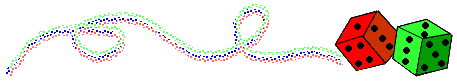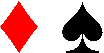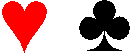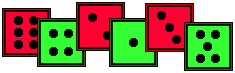Objective 15 Calculate theoretical probability, using numbers between 0 and 1.
Problem
 The theoretical probability of rolling a 1 is 0.125, of rolling a 2 is 0.25, of rolling a 3 is 0.25 and of rolling a 5 is 0.375. You can only roll a 1, 2, 3 or 5 on this die.How many sides are there? How many sides have a 1? a 2? a 3? a 5?
Manipulative
 Materials: deck of cards (2 through 10 in all suits). Draw five or more cards from the deck (up to 10 cards in all) and lay them out on a table. Construct a spinner which has an equal number of spaces as there are cards. Write the number for each card on one of the spaces in the spinner. Calculate the theoretical probability for each type of card, i.e., for each possible value. For example, calculate the theoretical probability of drawing or spinning a '2'. Construct an experiment to check the similarity of the experimental probabilities of the spinner and the cards.Game
 Materials: dice face cards. Play a game of rummy (2 or 3 players). Deal each player 6 cards from a well shuffled deck.Take turns drawing either from the discard pile or from the fresh deck, exchanging cards in your hand, until one player has the cards which represent the net for a 6-sided die which has: round 1: a probability of rolling a 1 as 0.5 round 2: a probability of rolling a 2 as 0.5 and of rolling a 3 as 0.5 round 3: an equal probability of rolling a 1, 2, 3, 4, 5 and 6. round 4: a probability of 0.333 for each of the even numbers 2 through 6. round 5: the sum of the faces as 21. round 6: a probability of rolling a 1 as 0.167, of rolling a 3 as 0.5 and of rolling a 5 as 0.333.